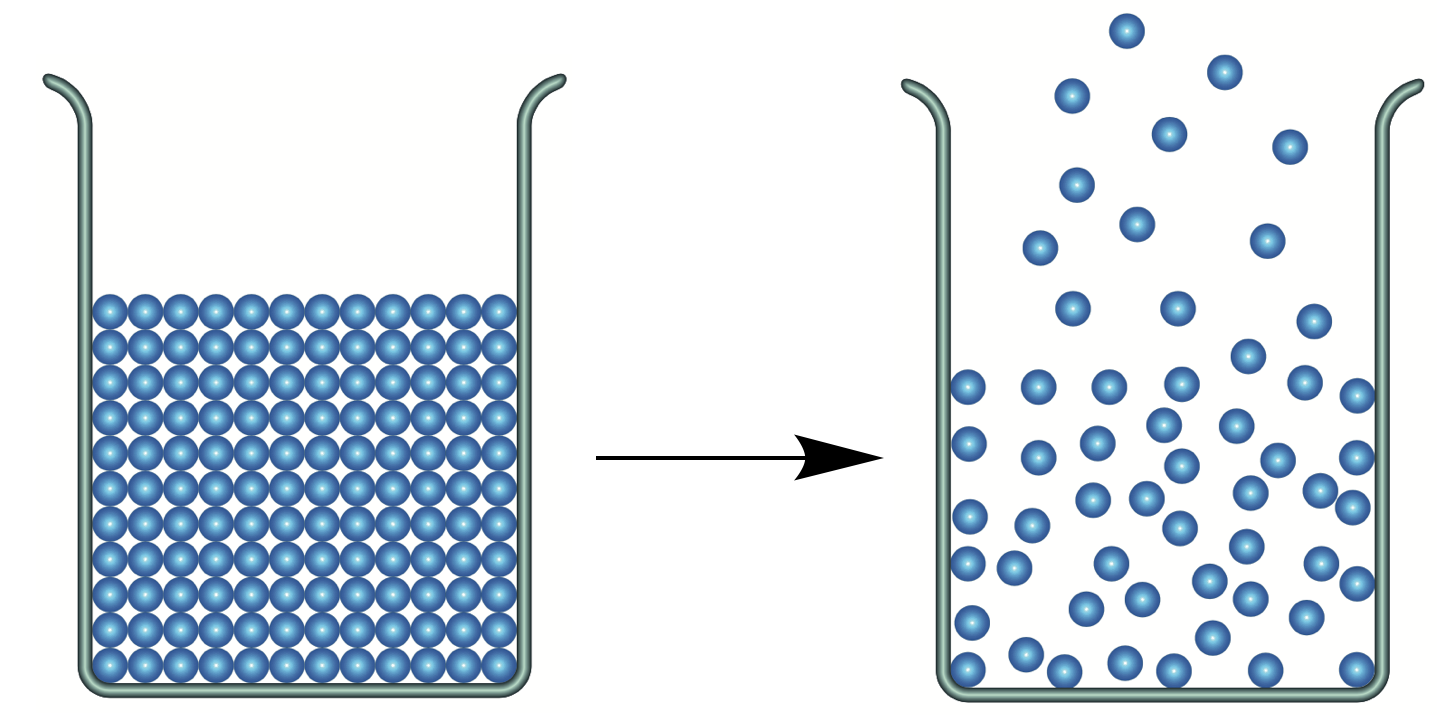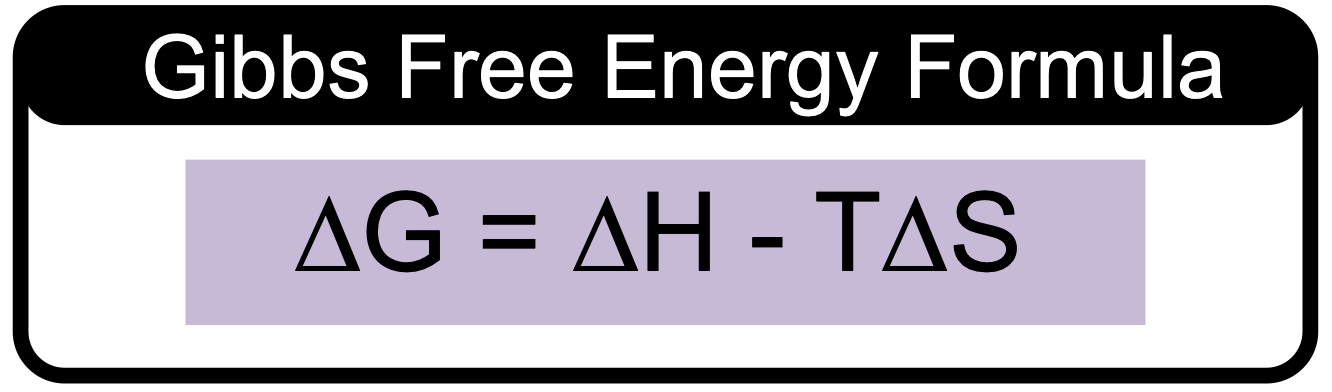Clutch Prep is now a part of Pearson
Ch.7 Energy, Rate and EquilibriumWorksheetSee all chapters

# Gibbs Free Energy (Simplified)

See all sections
Sections
Nature of Energy
First Law of Thermodynamics
Endothermic & Exothermic Reactions
Bond Energy
Thermochemical Equations
Heat Capacity
Thermal Equilibrium (Simplified)
Hess's Law
Rate of Reaction
Energy Diagrams
Chemical Equilibrium
The Equilibrium Constant
Le Chatelier's Principle
Solubility Product Constant (Ksp)
Spontaneous Reaction
Entropy (Simplified)
Gibbs Free Energy (Simplified)

Gibbs Free Energy represents energy associated with a chemical reaction that can be used to do work.

###### Gibbs Free Energy

Concept #1: Gibbs Free Energy (∆G)

Example #1: If ∆G is small and positive, which of the following statements is true?

Concept #2: Predicting Spontaneity

Example #2: PCl3 (g) + Cl2 (g) ⇌ PCl5 (g) At 25°C, ΔH° = - 92.50 kJ. Which of the following statements is(are) true?

Practice: What are the signs of ∆H, ∆S and ∆G for the spontaneous conversion of a solid into gas?Practice: Consider the combustion of butane gas and predict the signs of ΔS, ΔH and ∆G.

C4H10(g) + 13/2 O2(g) ⟶ 4 CO2(g) + 5 H2O(g)

Concept #3: Calculations of Gibbs Free EnergyExample #3: For a particular reaction, ΔH = - 111.4 kJ and ΔS = - 25.0 J/K.

Calculate ΔG for this reaction at 298º K. What can be said about the spontaneity of the reaction at 298º K?

Practice: A particular reaction has ΔG = –350 kJ and ΔS = –350 J/K at 24°C. How much heat will be released/absorbed?

Practice: For a reaction in which ΔH = 125 kJ and ΔS = 325 J/K, determine the temperature in Celsius above which the reaction is spontaneous.